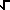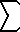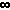Dave's Math Tables: Expansions for PI(Math | OddsEnds | Constants | PI)

Discoverer: Archimedes (287-212 BC) Found 3 10/17 < PI < 3 1/7PI = 3. 1415926535 8979323846 2643383279 5028841971 6939937510 5820974944 5923078164 0628620899 8628034825 3421170679 ...

Vieta's Formula

2/PI =2/2 *( 2 +2 )/2 *(2 + (( 2 +2) ) ) * ...c

Leibnitz's Formula

PI/4 = 1/1 - 1/3 + 1/5 - 1/7 + ...

Wallis Product

PI/2 = 2/1 * 2/3 * 4/3 * 4/5 * 6/5 * 6/7 * ...

2/PI = (1 - 1/22)(1 - 1/42)(1 - 1/62)...

Lord Brouncker's Formula

```4/PI = 1 +        1
----------------
2 +     32
------------
2 +   52
---------
2 + 72 ...
```

(PI2)/8 = 1/12 + 1/32 + 1/52 + ...

(PI2)/24 = 1/22 + 1/42 + 1/62 + ...

(PI2)/6 =(n = 1..) 1/n2 = 1/12 + 1/22 + 1/32 + ...

(or more generally...)(n = 1..) 1/n(2k) = (-1)(k-1) PI(2k) 2(2k) B(2k) / ( 2(2k)!)

B(k) = the k th Bernoulli number. eg. B0=1 B1=-1/2 B2=1/6 B4=-1/30 B6=1/42 B8=-1/30 B10=5/66. Further Bernoulli numbers are defined as (n 0)B0 + (n 1)B1 + (n 2)B2 + ... + (n (n-1))B(N-1) = 0 assuming all odd Bernoulli #'s > 1 are = 0. (n k) = binomial coefficient = n!/(k!(n-k)!)

See Power Summations #2 for simplified expressions (without the Bernoulli notation) of these sums for given values of k.